Miscellaneous

Chapter 2 Class 11 Relations and Functions (Term 1)
Serial order wise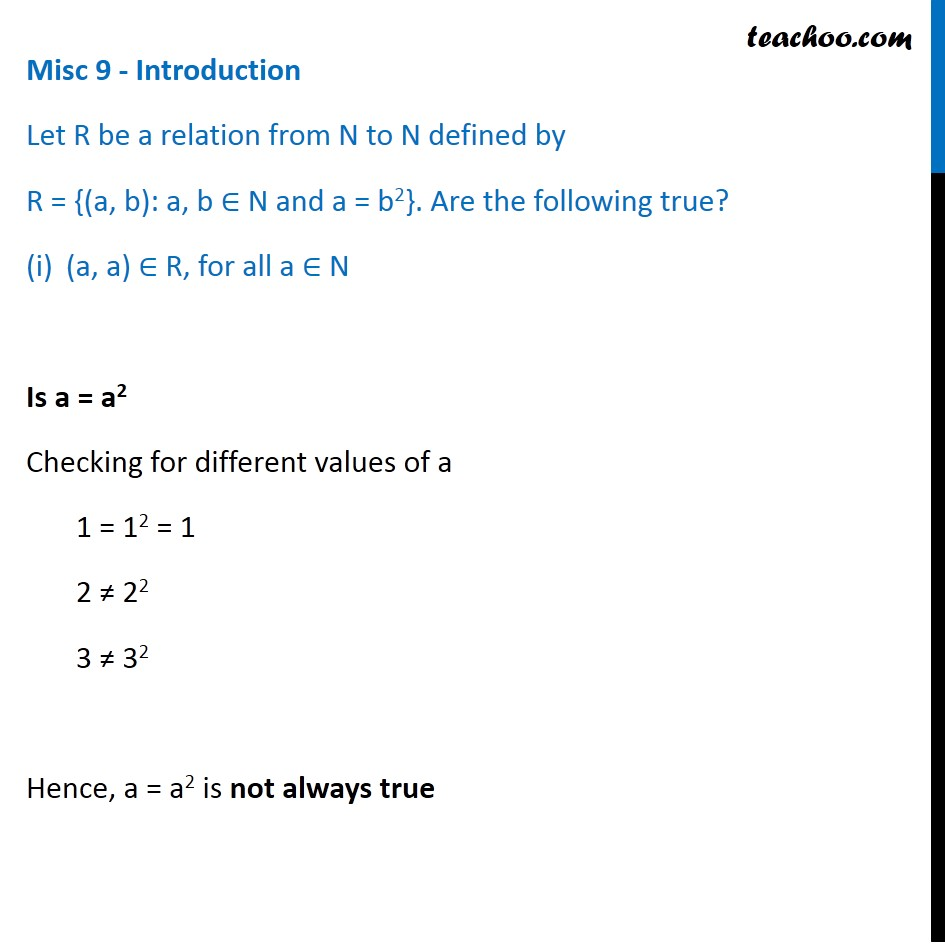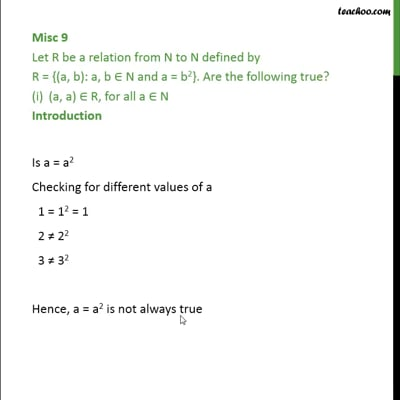This video is only available for Teachoo black users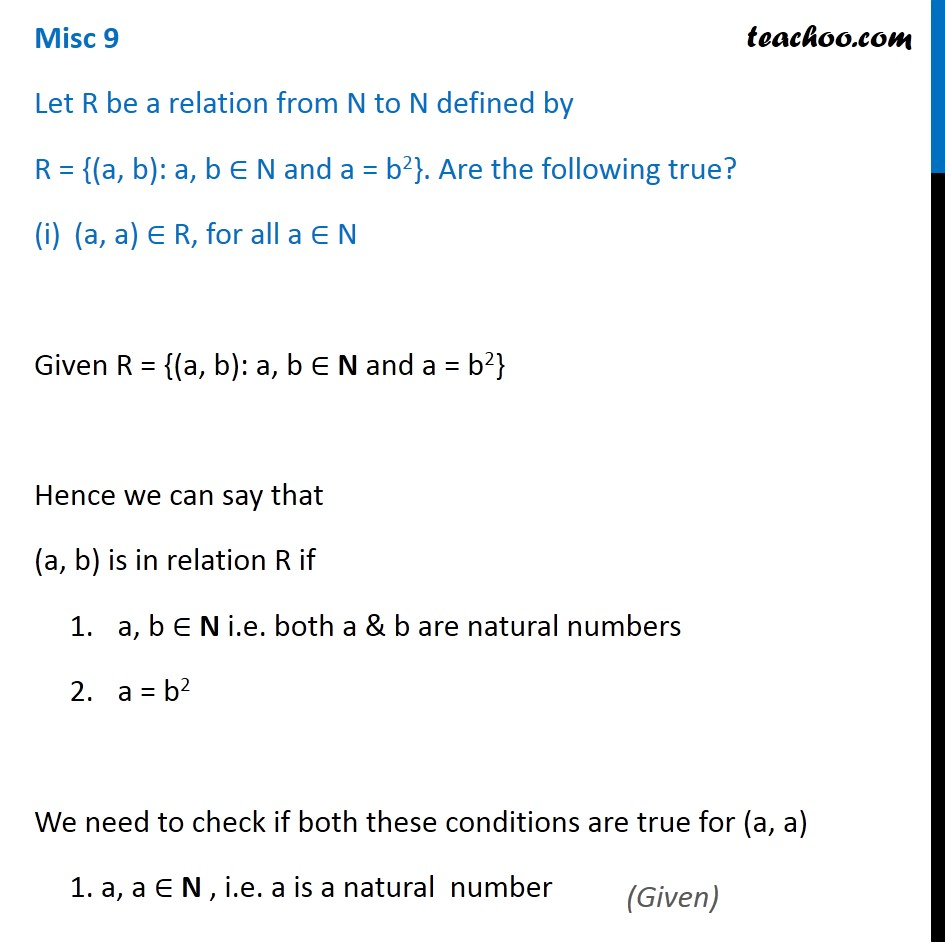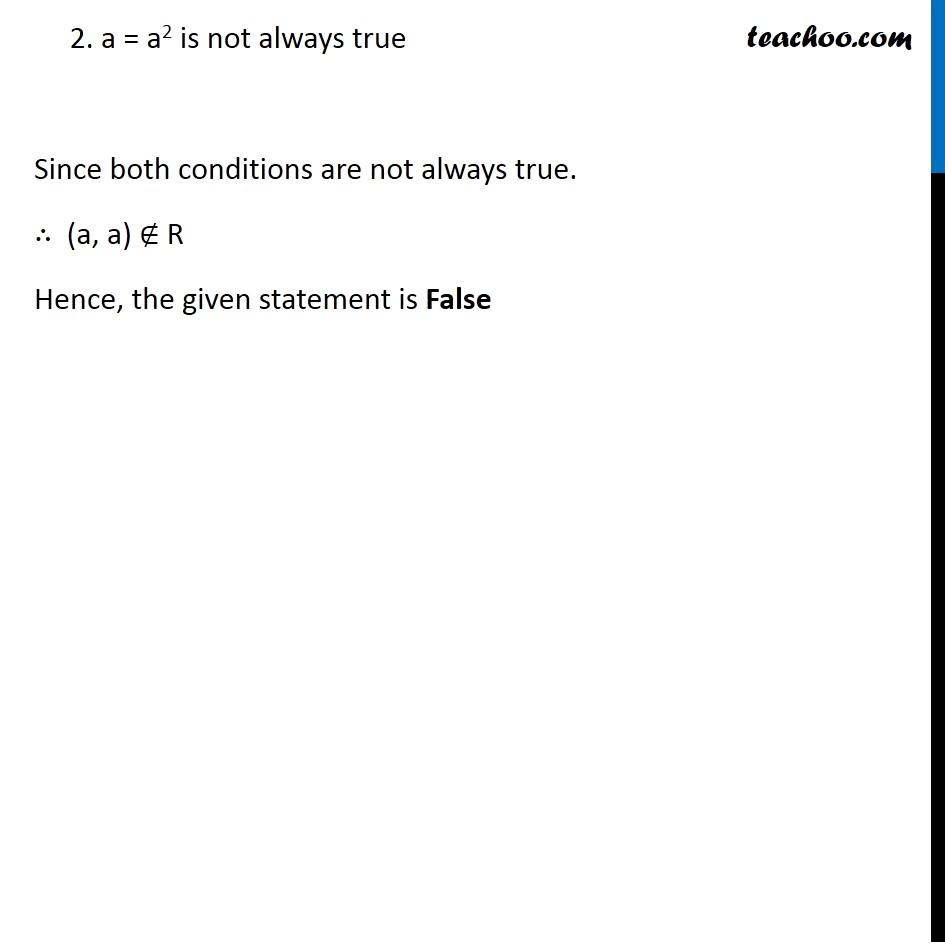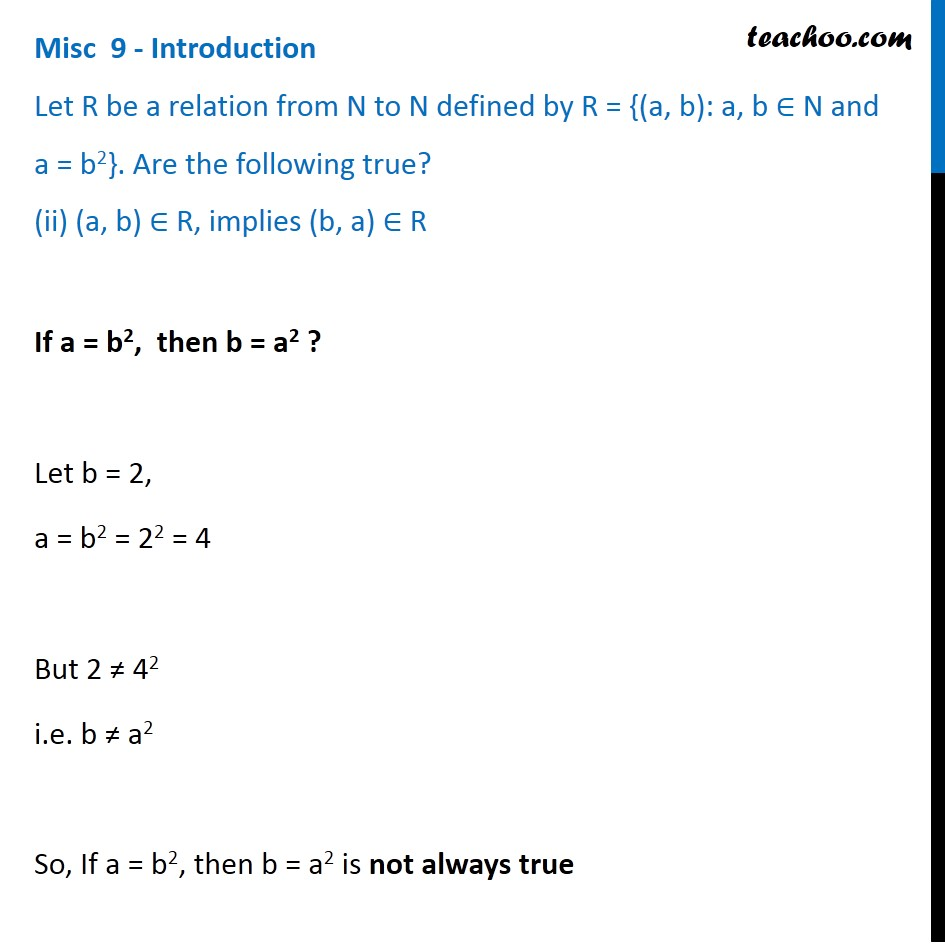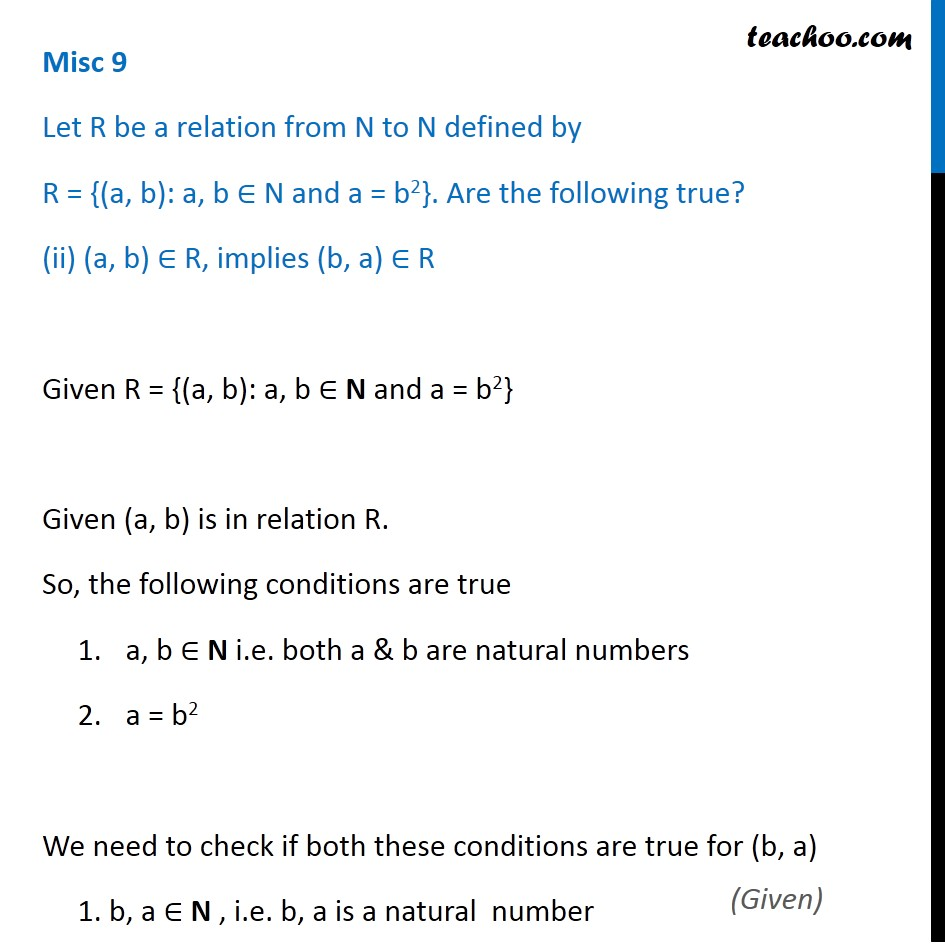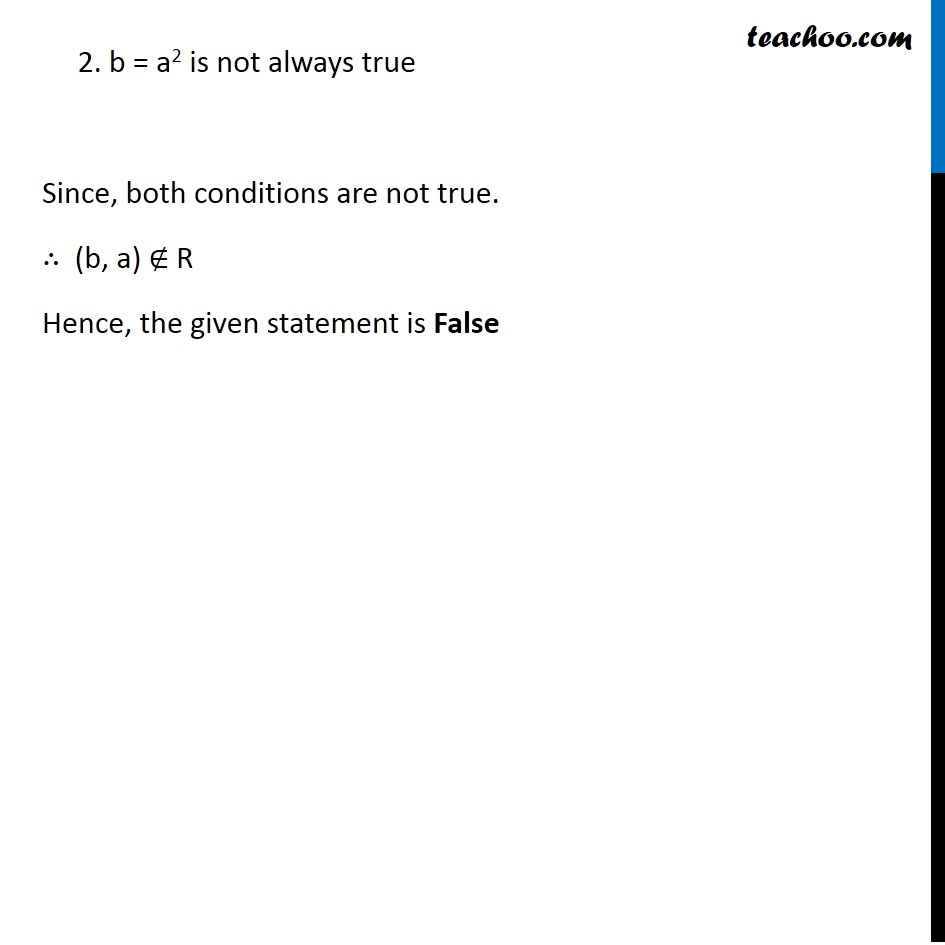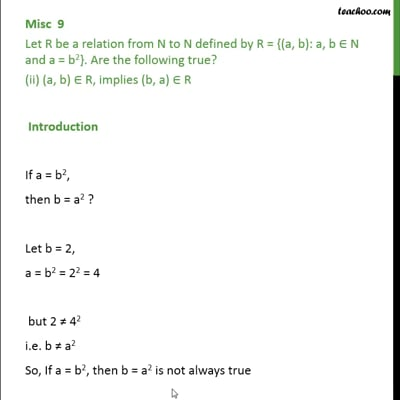This video is only available for Teachoo black users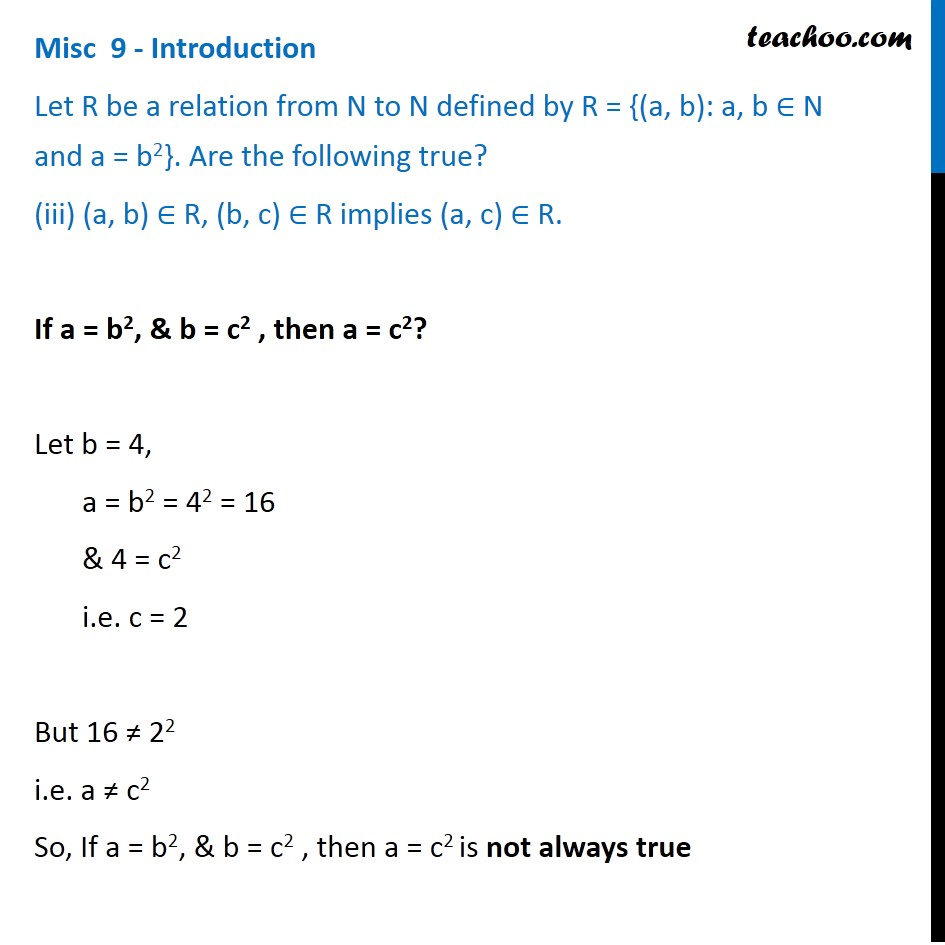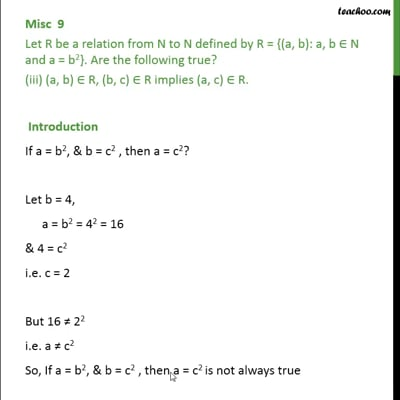This video is only available for Teachoo black users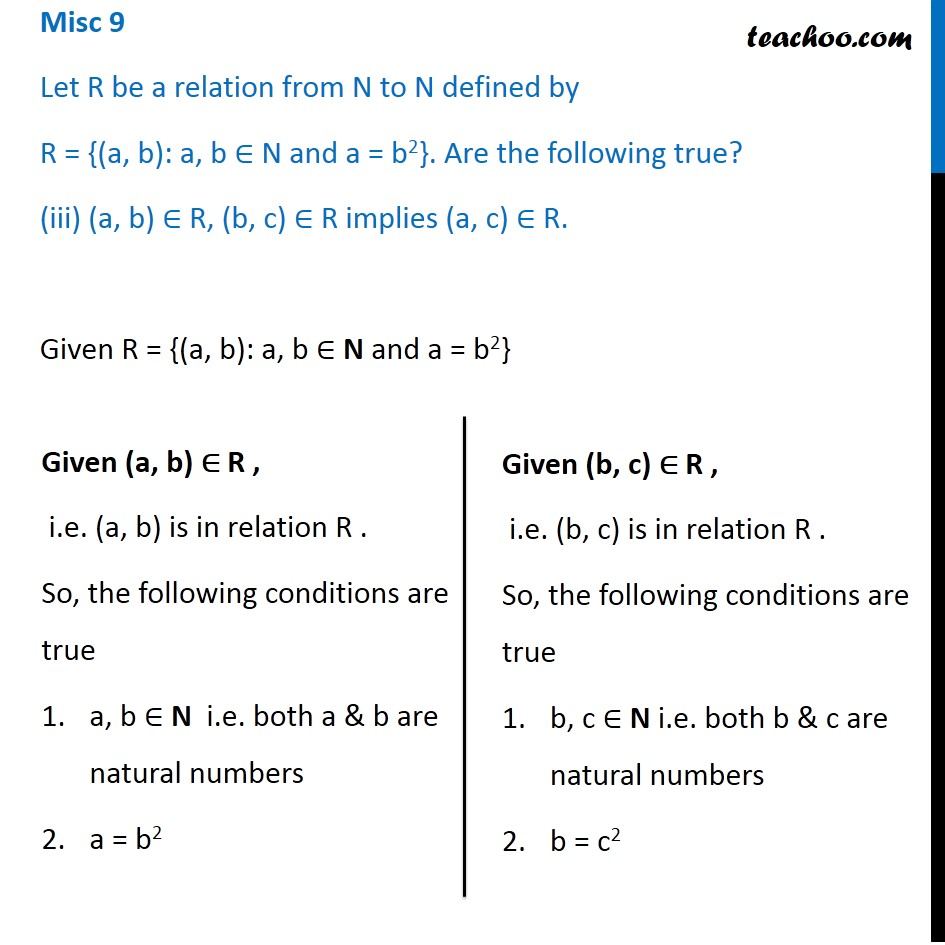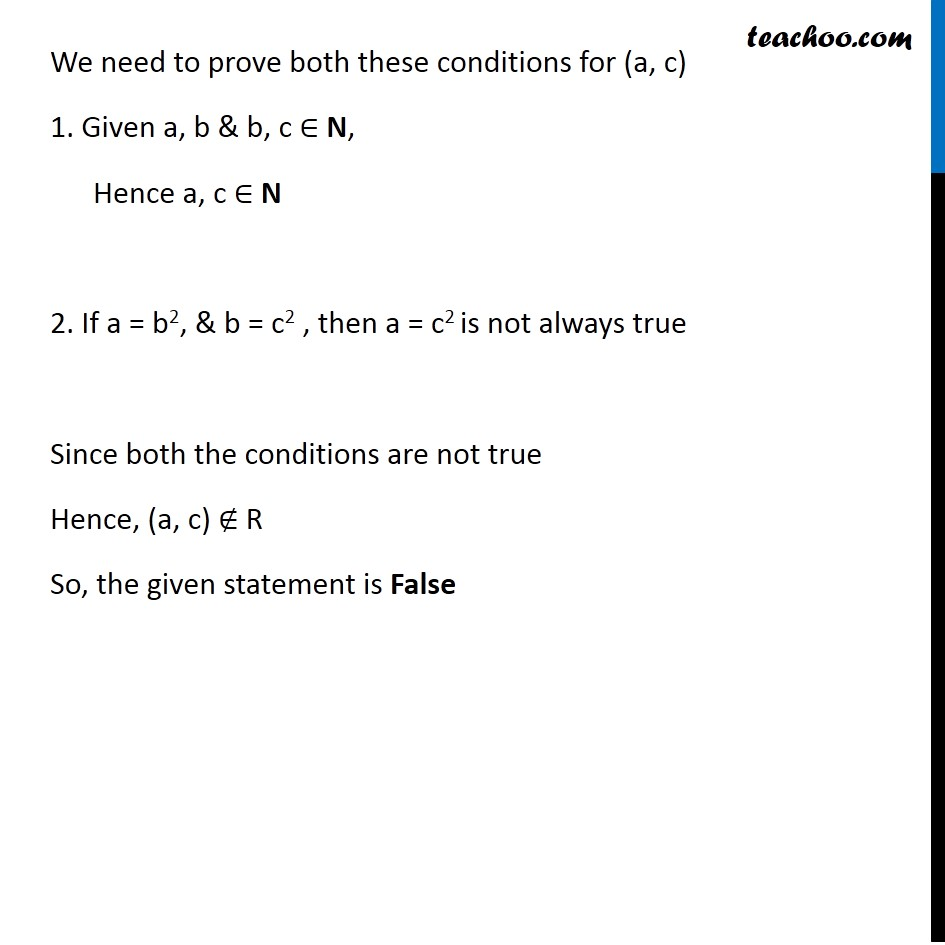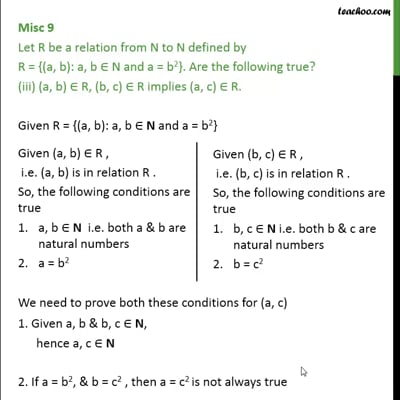This video is only available for Teachoo black users

### Transcript

Misc 9 - Introduction Let R be a relation from N to N defined by R = {(a, b): a, b ∈ N and a = b2}. Are the following true? (a, a) ∈ R, for all a ∈ N Is a = a2 Checking for different values of a 1 = 12 = 1 2 ≠ 22 3 ≠ 32 Hence, a = a2 is not always true Misc 9 Let R be a relation from N to N defined by R = {(a, b): a, b ∈ N and a = b2}. Are the following true? (a, a) ∈ R, for all a ∈ N Given R = {(a, b): a, b ∈ N and a = b2} Hence we can say that (a, b) is in relation R if a, b ∈ N i.e. both a & b are natural numbers a = b2 We need to check if both these conditions are true for (a, a) 1. a, a ∈ N , i.e. a is a natural number 2. a = a2 is not always true Since both conditions are not always true. ∴ (a, a) ∉ R Hence, the given statement is False Misc 9 - Introduction Let R be a relation from N to N defined by R = {(a, b): a, b ∈ N and a = b2}. Are the following true? (ii) (a, b) ∈ R, implies (b, a) ∈ R If a = b2, then b = a2 ? Let b = 2, a = b2 = 22 = 4 But 2 ≠ 42 i.e. b ≠ a2 So, If a = b2, then b = a2 is not always true Misc 9 Let R be a relation from N to N defined by R = {(a, b): a, b ∈ N and a = b2}. Are the following true? (ii) (a, b) ∈ R, implies (b, a) ∈ R Given R = {(a, b): a, b ∈ N and a = b2} Given (a, b) is in relation R. So, the following conditions are true a, b ∈ N i.e. both a & b are natural numbers a = b2 We need to check if both these conditions are true for (b, a) 1. b, a ∈ N , i.e. b, a is a natural number 2. b = a2 is not always true Since, both conditions are not true. ∴ (b, a) ∉ R Hence, the given statement is False Misc 9 - Introduction Let R be a relation from N to N defined by R = {(a, b): a, b ∈ N and a = b2}. Are the following true? (iii) (a, b) ∈ R, (b, c) ∈ R implies (a, c) ∈ R. If a = b2, & b = c2 , then a = c2? Let b = 4, a = b2 = 42 = 16 & 4 = c2 i.e. c = 2 But 16 ≠ 22 i.e. a ≠ c2 So, If a = b2, & b = c2 , then a = c2 is not always true Misc 9 Let R be a relation from N to N defined by R = {(a, b): a, b ∈ N and a = b2}. Are the following true? (iii) (a, b) ∈ R, (b, c) ∈ R implies (a, c) ∈ R. Given R = {(a, b): a, b ∈ N and a = b2} Given (a, b) ∈ R , i.e. (a, b) is in relation R . So, the following conditions are true a, b ∈ N i.e. both a & b are natural numbers a = b2 Given (b, c) ∈ R , i.e. (b, c) is in relation R . So, the following conditions are true b, c ∈ N i.e. both b & c are natural numbers b = c2 We need to prove both these conditions for (a, c) 1. Given a, b & b, c ∈ N, Hence a, c ∈ N 2. If a = b2, & b = c2 , then a = c2 is not always true Since both the conditions are not true Hence, (a, c) ∉ R So, the given statement is False